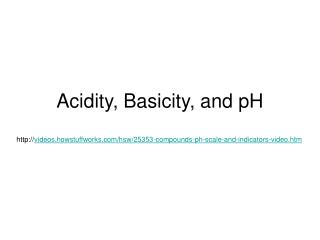DownloadDownload PresentationAcidity, Basicity, and pH

# Acidity, Basicity, and pH

Download Presentation## Acidity, Basicity, and pH

- - - - - - - - - - - - - - - - - - - - - - - - - - - E N D - - - - - - - - - - - - - - - - - - - - - - - - - - -
##### Presentation Transcript

1. Acidity, Basicity, and pH http://videos.howstuffworks.com/hsw/25353-compounds-ph-scale-and-indicators-video.htm

2. Self-Ionization of Water • Water acts as both acid and base in same reaction • Proceeds minimally to the right • H2O (l) + H2O (l) H3O+(aq) + OH-(aq) • Pure H2O, 25°C, [H3O+] & [OH-]=1.0x10-7 • Concentration is small but significant • Keq = [H3O+][OH-]/[H2O]2

3. Self-Ionization of Water • Pure water and dilute solutions [H2O] same-[H2O] moved to left side • Keq [H2O]2=[H3O+][OH-] • Kw = [H3O+][OH-] ion product constant • Pure water, 25°C, Kw = 1.0 x 10-14 • Kw = (1.0 x 10-7)(1.0 x 10-7) • Applies for all water solutions at 25°C, even when [H3O+][OH-] are not equal

4. Self-Ionization of Water • Acidic, basic and neutral solutions all contain H3O+ and OH- • Acidic – [H3O+] > 10-7 • Basic – [OH-] > 10-7 • If the concentration of H3O+ in blood is 4.0x10-8 M, is blood acidic, basic, or neutral? What is the concentration of OH- ions in blood?

5. Self-Ionization of Water • Blood is basic, [H3O+] 4.0x10-8 < 1.0x10-7 • Kw = [H3O+][OH-] • 1.0x10-14 = (4.0x10-8)[OH-] • [OH-] = 2.5x10-7 M

6. Example • What is the concentration of H3O+ ions in a wheat flour and water solution if [OH-] = 1.0x10-8 M? Is wheat flour and water acidic, basic, or neutral? • 1.0 x 10-14 = [H3O+](1.0 x 10-8 M) • [H3O+] = 1.0 x 10-6 M • Acidic

7. pH Scale • Based on logarithms pH = -log [H3O+] • Number’s base 10 logarithm is its exponent when 10 is the base, log (0.001) = 3 10-3 = 0.001 • 1 unit change in pH is 10-fold change in concentration of H3O+ • Sig. Figs. – number of digits after decimal should equal number of sig. figs. In original number

8. pH • In one brand of vegetable juice, the concentration of H3O+ ions is 7.3 x 10-5 M What is the pH of the juice? • pH = -log [H3O+] • pH = - log (7.3 x 10-5) • Using log key = -4.13667714 • pH = -(-4.13667714) = 4.14

9. Example • In household bleach, the concentration of OH- ions is 5.0 x 10-2 M. What is the pH? • 1.0 x 10-14 = [H3O+](5.0 x 10-2) • [H3O+] = 2.0 x 10-13 • pH = -log (2.0 x 10-13) • pH = 12.70

10. Measuring pH • Use acid-base indicator • Indicator equation • HIn (aq) + H2O (l) H3O+ (aq) + In- (aq) clear red • Add acid, shift equilibrium to left • (litmus – HIn red) • Add base, shift equilibrium to right • (litmus – In-blue)

11. Measuring pH • Each indicator has a different equilibrium constant • Different pH range over which color changes • Select indicator depending on pH range